# Review: Semi-Supervised Learning with Ladder Networks

## Ladder Network, Γ-Model: Minimize Cost of Latent Features

Semi-Supervised Learning with Ladder Networks
Ladder Network, Γ-Model, by The Curious AI Company, Nokia Labs, and Aalto University, 2015 NIPS, Over 1200 Citations (Sik-Ho Tsang @ Medium)
Semi-Supervised Learning, Image Classification

• The proposed model is trained to simultaneously minimize the sum of supervised and unsupervised cost functions, built on the top of the Ladder Network.

# Outline

1. Minimizing Deep Features
2. Ladder Network & Γ-Model
3. Experimental Results

# 1. Minimizing Deep Features

## 1.1. Denoising Autoencoder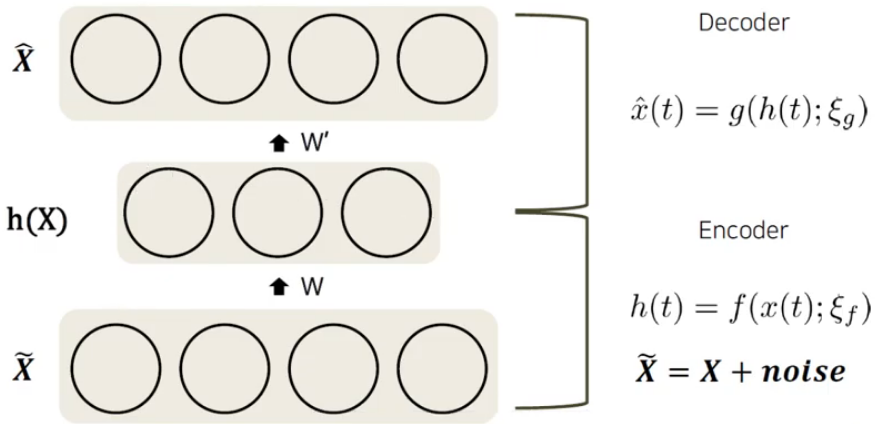Denoising Autoencoder (Figure from a Korean Language Presentation)
• In Denoising Autoencoder, noise is added into the clean input x, to become ~x. ~x is input to autoencoder. Then, the autoencoder is trying to reconstruct ^x which is as close as x.
• By doing so, the deep latent feature at the middle has rich feature information which can be used for fine-tuning on other datasets.
• To train the Denoising Autoencoder, the cost is to minimize the reconstructed output ^x and clean input x:
• However, there is no cost function to minimize the difference of the latent feature at the middle.

## 1.2. Minimizing Deep Feature Difference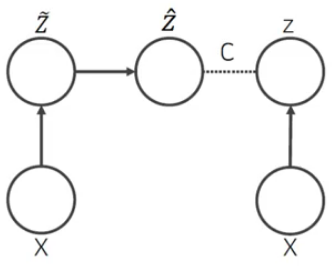Minimizing Deep Features (Figure from a Korean Language Presentation)
• One way is to directly minimize the deep feature difference of z. The cost function is identical to that used in a Denoising Autoencoder except that latent variables z replace the observations x:

# 2. Ladder Network & Γ-Model

• (Here, only the conceptual idea is presented.)

## 2.1. Ladder Network

• x is the image input and y is the output which can be the label.

The clean path is the standard supervised learning path.

The corrupted path is the path that the noise is added every layer to corrupt the feature signals. And it try to predict the label y as well.

The denoising path is to reconstruct x, with the help of features at the corrupted path. Every layer contributes to the cost function:

• Since the cost function needs both the clean z(l) and corrupted ˜z(l), during training, the encoder is run twice: a clean pass for z(l) and a corrupted pass for ˜z(l).
• g is a denoising function with the inputs, from the previous layer and also from the corresponding layer at the corrupted path.

## 2.2. Γ-Model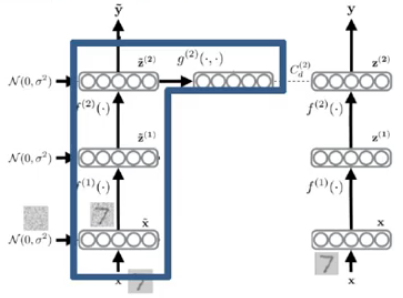Γ-Model (Figure from a Korean Language Presentation)
• Γ-Model is the simple special case of the Ladder Network.
• This corresponds to a denoising cost only on the top layer and means that most of the decoder can be omitted.

# 3. Experimental Results

## 3.1. Fully Connected MLP on MNIST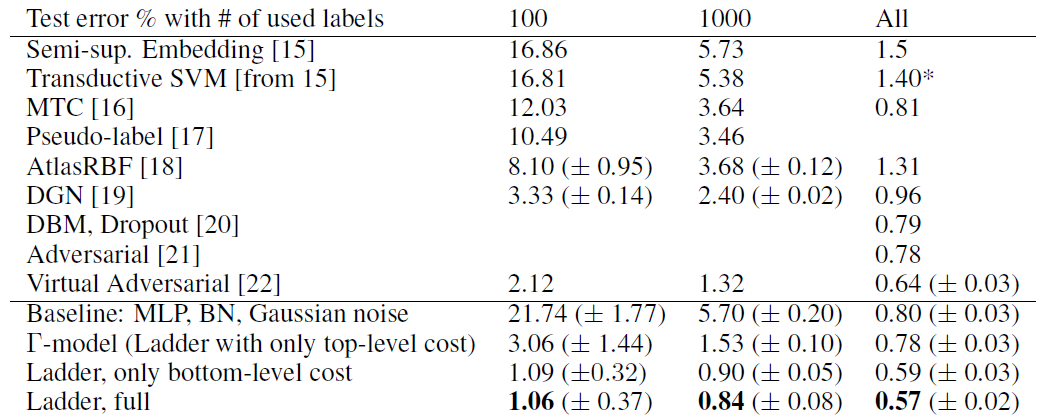MNIST test errors in the permutation invariant setting
• The baseline MLP model is 784–1000–500–250–250–250–10.

The proposed method outperforms all the previously reported results., e.g.: Pseudo-Label (PL).

• Encouraged by the good results, we also tested with N=50 labels and got a test error of 1.62 %.

The simple Γ-Model also performed surprisingly well, particularly for N=1000 labels.

• With N=100 labels, all models sometimes failed to converge properly.

## 3.2. CNN on MNIST

• 2 models, Conv-FC and Conv-Small using Γ-Model, are used.

More convolutions improve the Γ-Model significantly although the variance is still high. The Ladder network with denoising targets on every level converges much more reliably.

## 3.3. CNN on CIFAR-10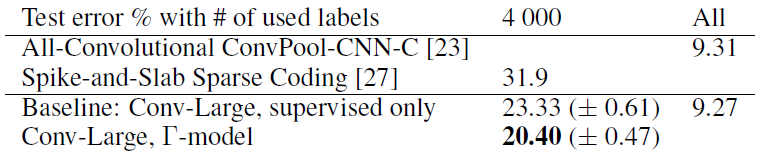Test results for CNN on CIFAR-10 dataset without data augmentation

With Conv-Large using Γ-Model, about 3% further of error rate is reduced when using N=4000 labels.

## References

[2015 NIPS] [Ladder Network, Γ-Model]
Semi-Supervised Learning with Ladder Networks

## Pretraining or Weakly/Semi-Supervised Learning

2013 [Pseudo-Label (PL)] 2015 [Ladder Network, Γ-Model] 2016 [Sajjadi NIPS’16] 2017 [Mean Teacher] 2018 [WSL] 2019 [Billion-Scale] [Label Propagation] [Rethinking ImageNet Pre-training] 2020 [BiT] [Noisy Student] [SimCLRv2]

--

--